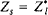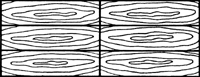# matching

(redirected from Perfect matching)
Also found in: Dictionary, Thesaurus, Medical, Financial.
Related to Perfect matching: complete matching

## matching

(impedance matching) Arranging electrical impedances so that maximum power is transferred from one device to another. This occurs when the input impedance of one device equals the output impedance of the other to which it is connected. See also feeder.
Collins Dictionary of Astronomy © Market House Books Ltd, 2006

## Matching

Used to describe the arrangement of timber veneers, such as book-matching, slip-matching and quarter-matching.
The following article is from The Great Soviet Encyclopedia (1979). It might be outdated or ideologically biased.

## Matching

in electrical circuit design, the practice that ensures the transmission of electromagnetic energy and signals with the lowest possible reflection factor, signal loss, and distortion. Matching consists in the proper choice of impedances for the source, transmission line, and load. Line and load are ideally matched when the characteristic impedance of the transmission line p is equal to the impedance of the load Zl = Rl + jXl or when Rl = ρ and Xl = 0, where Rl is the active part of the impedance and Xl is the reactive part. Under these circumstances, traveling waves characterized by a standing-wave ratio of unity are established in the transmission line.

For lines with negligibly small electrical losses, matching—and, consequently, the maximum effective transfer of energy from the source to the load—is achieved when the impedance of the source Zs and the load Z, are complex conjugates, that is, whenor Rs = ρ = Rl and Xs = – Xl. In this case, the reactive impedance of the circuit is zero, and the conditions for resonance are satisfied. Such conditions enable electrical systems to operate with better efficiency, for example, with improved use of frequency ranges, better interference rejection, and reduced frequency distortion of radio signals.

Matching is especially important in microwave engineering, where it can be achieved by means of the following: impedance-matching transformers, such as stubs or dielectric sleeves inserted in the transmission line or sections of transmission lines with specially chosen characteristic impedances and lengths; various matching devices that compensate the reactive components of the impedance, such as stubs, phase shifters, and irises, placed in the transmission line; absorbing loads; directional couplers; and various devices for converting from one mode of transmission to another, such as coaxial-waveguide couplers, baluns, and flexible and rotating connections.

The degree of matching can be assessed by measuring the reflection factor and the standing-wave ratio. In practice, matching is considered optimum when the standing-wave ratio in the chosen frequency range does not exceed 1.2 or 1.3 (1.05 for measuring instruments). In some cases, the response of source parameters, such as frequency, power, and noise level, to changes in load, the occurrence of electrical breakdowns in the line, and heating in some sections of the line can serve as indirect indicators of matching.

### REFERENCES

Fizicheskie osnovy elektrotekhniki. Edited by K. M. Polivanov. Moscow-Leningrad, 1950.
Valitov, R. A., and V. N. Sretenskii. Radiotekhnicheskie izmereniia. Moscow, 1970.
Lebedev, I. V. Tekhnika i pribory SVCh, 2nd ed., vol. 1. Moscow, 1970.
Gonorovskii, I. S. Radiotekhnicheskie tsepi i signaly, 2nd ed. Moscow, 1971.

V. N. SRETENSKII

## matching

[′mach·iŋ]
(computer science)
A computer problem-solving method in which the current situation is represented as a schema to be mapped into the desired situation by putting the two in correspondence.
(electricity)
Connecting two circuits or parts together with a coupling device in such a way that the maximum transfer of energy occurs between the two circuits, and the impedance of either circuit will be terminated in its image.
(mathematics)
A set of edges in a graph, no two of which have a vertex in common. Also known as independent edge set.
The bringing of two or more signals or indications into suitable position or condition preliminary to making a measurement, as on a loran indicator or a sky compass.
McGraw-Hill Dictionary of Scientific & Technical Terms, 6E, Copyright © 2003 by The McGraw-Hill Companies, Inc.

## matchingmatching, 2
A system of matchboards, or of sheets of wood veneer, arranged to emphasize grain pattern, as in book matching or herringbone matching.
McGraw-Hill Dictionary of Architecture and Construction. Copyright © 2003 by McGraw-Hill Companies, Inc.
References in periodicals archive ?
Since |[L.sub.u] - {a, b}| = |[R.sub.u]|, [M.sub.u] is a perfect matching in [P.sub.u].
Claim 1: For each i, where 0 [less than or equal to] i < L, [M.sub.i] is a plane perfect matching in P.
A graph G is matching persistent if by removing any perfect matching M from G, the resulting graph, G - M, has a perfect matching.
by removing an arbitrary set of k - 1 edge-disjoint perfect matchings from G, the resulting graph still contains a perfect matching, and
We showed in Section 5 that [P.sub.q][][K.sub.4] has a perfect matching that cannot be extended to a perfect matching when q [greater than or equal to] 3.
From the definition it is readily apparent that the graphs are Hamiltonian for d [greater than or equal to] 2 and admit perfect matchings for d [greater than or equal to] 1.
Haggkvist studied extendability of perfect matchings in graphs with p vertices (p even) satisfying the Ore-type condition that the sum of degrees of any two non-adjacent vertices is at least p+1.
(Note, when q = 4, the only matchings that produce cuts in [K.sub.m][][C.sub.4] are those corresponding to perfect matchings in [C.sub.4] and hence both resulting components are even.) But then we extend the cut to a pairing.
For a partition of E(G) into three perfect matchings [M.sup.1.sub.G], [M.sup.2.sub.G], [M.sup.3.sub.G], observe that the edges of the triangle [a.sub.1][a.sub.2][a.sub.3] belong to different sets [M.sup.i.sub.G].
Moreover, in the definition we maintain the following property: for every non-root node with label W in T the set [[epsilon].sub.G](W) has three elements, and they belong to different sets in the partition of E(G) into three perfect matchings. We denote the edge of [[epsilon].sub.G](W) that belongs to [M.sup.i.sub.G] by [e.sup.i.sub.W].
That is, we partition the edge set E([K.sub.4]) into perfect matchings [MATHEMATICAL EXPRESSION NOT REPRODUCIBLE IN ASCII].
Let us now check if the definition of T really maintains the aforementioned property that for every non-root node with label [W.sub.T] in T, [[epsilon].sub.G]([W.sub.T]) is a 3-edge-cut (i.e., a set of three edges, such that their removal disconnects the graph), and the edges belong to different perfect matchings in the partition [M.sup.1.sub.G], [M.sup.2.sub.G], [M.sup.3.sub.G].

Site: Follow: Share:
Open / Close